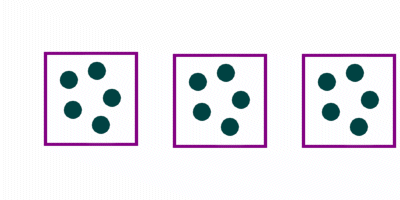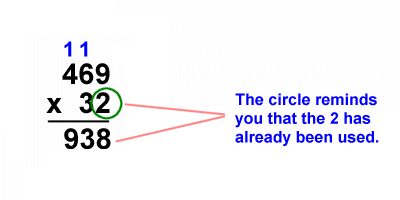# Kids Math

## Multiplication Tips and Tricks

There are a number of tips and tricks you can use that may help you with your multiplication. Different tricks help different people, so some of these may help you a lot, while others may not. Just try them out to see what works for you.

Draw a Picture

One of the simplest ways to understand multiplication is to draw a picture.

Example:

5 x 3 = ?Now you can just count up the dots to discover there are 15 total dots: 5 x 3 = 15.

Use Multiples to Find the Answer

Let's say you can't remember what 5 x 7 is, but you can remember that 5 x 5 = 25. Now you can just keep on adding 5's to 25: 25 + 5 = 30, 30 + 5 = 35, therefore 5 x 7 = 35.

You can learn a lot and work on your times tables by counting in multiples. Try it for the number 4: 4, 8, 16, 20, 24, 28, 32, ….

When Multiplying by the number....
• 2 - Remember that the answer will always be an even number. If your answer isn't even, then you need to try it again.
• 5 - The answer will always end in a 0 or a 5
• 10 - You just have to put a zero behind the other number. With 100 put two zeros.
• 11 - When multiplying 11 by numbers less than 10, you can just write the number twice for the answer. For example, 5 x 11 = 55, 8 x11 = 88
Break Apart the Number

Some numbers are easy to break apart and then add the two results. This is pretty much what we do when we do long multiplication, but you can do it on smaller problems if it makes them easier to solve.

Example:

1) 14 x 12 = ?

You may not have memorized 14 x 12, but you should know 7 x 12 if you learned the times table so you can do the following:

(2 x 7 x 12) = 2 x 84 = 84 + 84 = 168

2) 42 x 6 = ?

In this case we will take advantage of 10s multiplying. We don't know what 42 x 6 is off the top of our head, but we do know 4 x 6 and 2 x 6, we can use these numbers to solve the problem:

42 x 6 = (10 x 4 x 6) + (2 x 6) = (10 x 24) + 12 = 240 + 12 = 252

Long Multiplication

If you are having trouble with long multiplication, one idea is to circle the numbers you have already used. This way you won't accidentally use them again.

Example:Go to our long multiplication page for more on this subject.

Fun Trick when Multiplying Numbers by 9

This works when multiplying numbers up to 10 by the number 9.

1) Hold your hands out in front of you with your fingers straight
2) Now, for whatever number you are multiplying 9 by, lower that finger. For example, if it is 9 x 4, lower the fourth finger from the right.
3) Now look at your fingers. If it was 9 x 4, you have three fingers still up to the right of the finger you lowered and six fingers on the left. This is actually the answer! 9 x 4 = 36.
4) Try this for other numbers and see that it works. It even works for 1 and 10 because if it's 1x9 you have 09, which is the same as 9. If your last finger is down for 9 x 10 you have 9 and 0 fingers up. That's 90!

 Multiplication Intro to Multiplication Long Multiplication Multiplication Tips and Tricks Division Intro to Division Long Division Division Tips and Tricks Fractions Intro to Fractions Equivalent Fractions Simplifying and Reducing Fractions Adding and Subtracting Fractions Multiplying and Dividing Fractions Decimals Decimals Place Value Adding and Subtracting Decimals Multiplying and Dividing Decimals Statistics Mean, Median, Mode, and Range Picture Graphs Algebra Order of Operations Exponents Ratios Ratios, Fractions, and Percentages Geometry Polygons Quadrilaterals Triangles Pythagorean Theorem Circle Perimeter Surface Area Misc Basic Laws of Math Prime Numbers Roman Numerals Binary Numbers

Back to Kids Math

Back to Kids Study